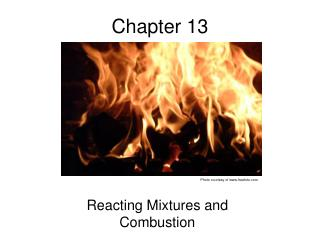Download PresentationChapter 13Chapter 13 - PowerPoint PPT Presentation

Download PresentationChapter 13
An Image/Link below is provided (as is) to download presentation

Download Policy: Content on the Website is provided to you AS IS for your information and personal use and may not be sold / licensed / shared on other websites without getting consent from its author. While downloading, if for some reason you are not able to download a presentation, the publisher may have deleted the file from their server.

- - - - - - - - - - - - - - - - - - - - - - - - - - - E N D - - - - - - - - - - - - - - - - - - - - - - - - - - -
Presentation Transcript

1. Chapter 13 Reacting Mixtures and Combustion Photo courtesy of www.freefoto.com

2. Fundamentals Chemical Equations: Modeling Air: • 79% Nitrogen, 21% Oxygen • Only the Oxygen reacts: Nitrogen considered inert • Mair = 28.97 kg/kmol or lb/lbmol (Tables A-1) Air Fuel Ratio:

3. Fundamentals • Common fuels modeled as simple hydrocarbons: • Natural Gas  Methane (CH4) • Gasoline  Octane (C8H18) • Diesel  Dodecane (C12H26) Chemical Equations: Stoichiometric Coefficients (Four equations and four unknowns) • Theoretical Air: • The minimum amount of air that provides the necessary oxygen • for complete combustion (i.e. For one mole of octane the • theoretical air is 59.5 moles)

4. Fundamentals • Percent Excess Air: • The percent of air supplied that is in excess of the theoretical air Example: Combustion of Octane with 50% excess air (or 150% theoretical) Stoichiometric Coefficients (Two equations and two unknowns)

5. Quiz One kg/min of methane is burned in a combustor with 25% excess air. The temperature and pressure of the air and fuel are 25oC and 101kPa respectively. The design velocity for each intake is 15 m/s. Determine the diameter of the air intake line in meters.

6. Quiz One kg/min of methane is burned in a combustor with 25% excess air. The temperature and pressure of the air and fuel are 25oC and 101kPa respectively. The design velocity for each intake is 15 m/s. Determine the diameter of the air intake line in meters. Theoretical Air • CH4+a(0.21O2+0.79N2)  bCO2+cH2O+dN2 • C) 1*1 = b*1 b=1 • 1*4 = c*2 c=2 • O) a*2*0.21 = b*2+c*1 a=9.524 • Theoretical air = 9.524 kmol(air)/kmol(fuel)

7. Quiz One kg/min of methane is burned in a combustor with 25% excess air. The temperature and pressure of the air and fuel are 25oC and 101kPa respectively. The design velocity for each intake is 15 m/s. Determine the diameter of the air intake line in meters. Actual Mass flow rate of air Actual Air = Theoretical * (1+%excess) = 9.524*1.25 = 11.9 kmol(air)/kmol(fuel)

8. Quiz One kg/min of methane is burned in a combustor with 25% excess air. The temperature and pressure of the air and fuel are 25oC and 101kPa respectively. The design velocity for each intake is 15 m/s. Determine the diameter of the air intake line in meters. Diameter of air intake

9. Enthalpy: Reacting Systems Tabular enthalpies inadequate due to arbitrary reference datums Standard Reference State (Stable Elements): Tref = 298.15 K, pref = 1 atm First Law:

10. Enthalpy: Reacting Systems Standard Reference State Tref = 25 oC Pref = 1 atm First Law:

11. Energy Balances: Steady State

12. Energy Balances: Steady State

13. Energy Balances: Steady State

14. Closed System Energy Balance

15. Fuel Enthalpies Enthalpy of Combustion For Example: A Control Volume at Steady State LHV (Lower Heating Value): The enthalpy of combustion when the reactants and products are at the standard reference state and the water formed by combustion is a gas HHV (Higher Heating Value): …water formed by combustion is a liquid

16. Adiabatic Flame Temperature When no power produced, and combustion carried out adiabatically, Tp reaches a theoretical maximum. When using tables, requires iteration to determine!

17. Adiabatic Flame Temperature

18. Fuel Cells Solid Oxide Fuel Cell Proton Exchange Membrane Fuel Cell

19. Third Law of Thermodynamics The absolute entropy of a pure-crystalline substance at the absolute zero of temperature is zero. Clip art courtesy of MS Office 2000 Ideal Gas

20. Entropy Balances Control Volumes at Steady State, Reacting System Closed, Reacting System

21. Chemical Exergy Thermo-mechanical Exergy found in Chapter 7 For a Hydrocarbon: CaHb

22. Chemical Exergy For Carbon Monoxide: CO For Water: H2O For N2, O2, CO2 For mixture of gas phases of Ideal Gases at T0, p0

23. Exergy Summary